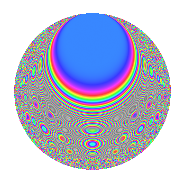# Properties

 Label 320.6.qLevel 320 Weight 6 Character orbit q Rep. character $$\chi_{320}(49,\cdot)$$ Character field $$\Q(\zeta_{4})$$ Dimension 116 Sturm bound 288

# Related objects

## Defining parameters

 Level: $$N$$ $$=$$ $$320 = 2^{6} \cdot 5$$ Weight: $$k$$ $$=$$ $$6$$ Character orbit: $$[\chi]$$ $$=$$ 320.q (of order $$4$$ and degree $$2$$) Character conductor: $$\operatorname{cond}(\chi)$$ $$=$$ $$80$$ Character field: $$\Q(i)$$ Sturm bound: $$288$$

## Dimensions

The following table gives the dimensions of various subspaces of $$M_{6}(320, [\chi])$$.

Total New Old
Modular forms 496 124 372
Cusp forms 464 116 348
Eisenstein series 32 8 24

## Trace form

 $$116q - 2q^{5} + O(q^{10})$$ $$116q - 2q^{5} + 4q^{11} + 4q^{15} - 2356q^{19} - 976q^{21} - 4q^{29} - 23056q^{31} + 3864q^{35} + 6734q^{45} + 220884q^{49} + 9472q^{51} - 14476q^{59} - 48084q^{61} - 27692q^{65} - 23296q^{69} + 33244q^{75} - 427312q^{79} - 551132q^{81} + 6248q^{85} + 231952q^{91} - 38420q^{95} - 406924q^{99} + O(q^{100})$$

## Decomposition of $$S_{6}^{\mathrm{new}}(320, [\chi])$$ into newform subspaces

The newforms in this space have not yet been added to the LMFDB.

## Decomposition of $$S_{6}^{\mathrm{old}}(320, [\chi])$$ into lower level spaces

$$S_{6}^{\mathrm{old}}(320, [\chi]) \cong$$ $$S_{6}^{\mathrm{new}}(80, [\chi])$$$$^{\oplus 3}$$

## Hecke characteristic polynomials

There are no characteristic polynomials of Hecke operators in the database